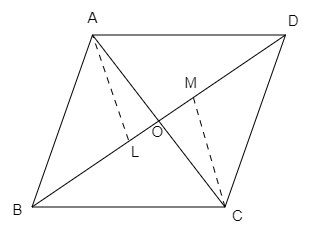"
">

# In the figure below, $\Delta A B C$ and $\Delta D B C$ are on the same base $B C$. If $A D$ and $B C$ intersect at $O$, prove that $\frac{\text { Area }(\Delta A B C)}{\text { Area }(\Delta D B C)}=\frac{A O}{D O}$"

Given:

$\Delta A B C$ and $\Delta D B C$ are on the same base $B C$.

$A D$ and $B C$ intersect at $O$.
To do:

We have to prove that  $\frac{\text { Area }(\Delta A B C)}{\text { Area }(\Delta D B C)}=\frac{A O}{D O}$.

Solution:

We know that,

Area of triangle $=\frac{1}{2}\times base \times height$

Therefore,

$\frac{ar(\triangle ABC)}{ar(\triangle DBC)}=\frac{\frac{1}{2}\times BC \times AL}{\frac{1}{2}\times BC \times DM}$

$=\frac{AL}{DM}$.....(i)

In $\triangle ALO$ and $\triangle CMO$,

$\angle LOA=\angle MOC$      (Vertically opposite angles)

$\angle ALO=\angle CMO=90^o$

Therefore,

$\triangle ALO \sim\ \triangle CMO$   (By AA similarity)

This implies,

$\frac{AL}{DM}=\frac{AO}{DO}$....(ii)    (Corresponding parts of similar triangle are proportional)

From equations (i) and (ii), we get,

$\frac{ar(\triangle ABC)}{ar(\triangle DBC)}=\frac{AO}{DO}$

Hence proved.

Updated on: 10-Oct-2022

18 Views